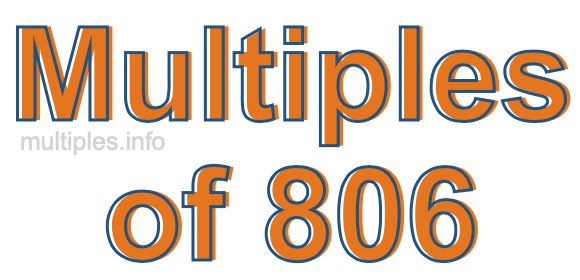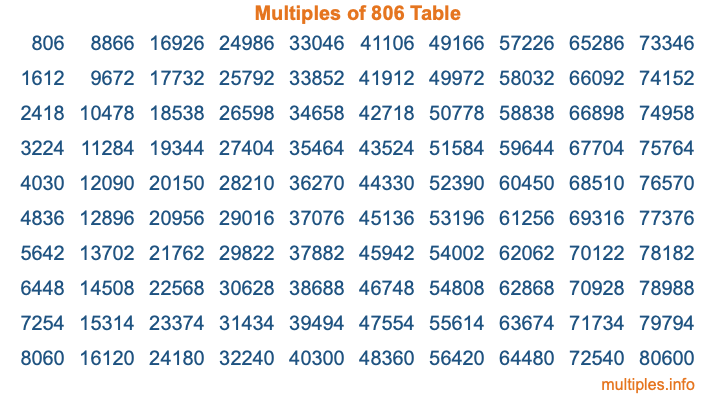Multiples of 806Welcome to the Multiples of 806 page. Here we will first teach you everything you will ever need to know about the multiples of 806, and then give you a study guide summary of everything we taught you to make sure you remember it all. Use this page to look up facts and learn information about the multiples of 806. This page will make you a multiples of eight hundred six expert!

Definition of Multiples of 806
Multiples of 806 are all the numbers that when divided by 806 equal an integer. Each of the multiples of 806 are called a multiple. A multiple of 806 is created by multiplying 806 by an integer.

Therefore, to create a list of multiples of 806, you start with 1 multiplied by 806, then 2 multiplied by 806, then 3 multiplied by 806, and so on for as long as you want. Thus, the list of the first five multiples of 806 is 806, 1612, 2418, 3224, and 4030. To see a larger list of multiples of 806, see the printable image of Multiples of 806 further down on this page. We also have a category where you can choose any nth multiple of 806.

Multiples of 806 Checker
The Multiples of 806 Checker below checks to see if any number of your choice is a multiple of 806. In other words, it checks to see if there is any number (integer) that when multiplied by 806 will equal your number. To do that, we divide your number by 806. If the the quotient is an integer, then your number is a multiple of 806.

Is  a multiple of 806?

Least Common Multiple of 806 and ...
A Least Common Multiple (LCM) is the lowest multiple that two or more numbers have in common. This is also called the smallest common multiple or lowest common multiple and is useful to know when you are adding our subtracting fractions. Enter one or more numbers below (806 is already entered) to find the LCM.

Check out our LCM Calculator if you need more details about the Least Common Multiple or if you need the LCM for different numbers for adding and subtraction fractions.

nth Multiple of 806
As we stated above, 806 is the first multiple of 806, 1612 is the second multiple of 806, 2418 is the third multiple of 806, and so on. Enter a number below to find the nth multiple of 806.

th multiple of 806

Multiples of 806 vs Factors of 806
806 is a multiple of 806 and a factor of 806, but that is where the similarities end. All postive multiples of 806 are 806 or greater than 806. All positive factors of 806 are 806 or less than 806.

Below is the beginning list of multiples of 806 and the factors of 806 so you can compare:

Multiples of 806: 806, 1612, 2418, 3224, 4030, etc.

Factors of 806: 1, 2, 13, 26, 31, 62, 403, 806

As you can see, the multiples of 806 are all the numbers that you can divide by 806 to get a whole number. The factors of 806, on the other hand, are all the whole numbers that you can multiply by another whole number to get 806.

It's also interesting to note that if a number (x) is a factor of 806, then 806 will also be a multiple of that number (x).

Multiples of 806 vs Divisors of 806
The divisors of 806 are all the integers that 806 can be divided by evenly. Below is a list of the divisors of 806.

Divisors of 806: 1, 2, 13, 26, 31, 62, 403, 806

The interesting thing to note here is that if you take any multiple of 806 and divide it by a divisor of 806, you will see that the quotient is an integer.

Multiples of 806 Table
Below is an image of the first 100 multiples of 806 in a table. The table is in chronological order, column by column. The first column has the first ten multiples of 806, the second column has the next ten multiples of 806, and so on.The Multiples of 806 Table is also referred to as the 806 Times Table or Times Table of 806. You are welcome to print out our table for your studies.

Negative Multiples of 806
Although not often discussed or needed in math, it is worth mentioning that you can make a list of negative multiples of 806 by multiplying 806 by -1, then by -2, then by -3, and so on, to get the following list of negative multiples of 806:

-806, -1612, -2418, -3224, -4030, etc.

Multiples of 806 Summary
Below is a summary of important Multiples of 806 facts that we have discussed on this page. To retain the knowledge on this page, we recommend that you read through the summary and explain to yourself or a study partner why they hold true.

There are an infinite number of multiples of 806.

A multiple of 806 divided by 806 will equal a whole number.

806 divided by a factor of 806 equals a divisor of 806.

The nth multiple of 806 is n times 806.

The largest factor of 806 is equal to the first positive multiple of 806.

806 is a multiple of every factor of 806.

806 is a multiple of 806.

A multiple of 806 divided by a divisor of 806 equals an integer.

806 divided by a divisor of 806 equals a factor of 806.

Any integer times 806 will equal a multiple of 806.

Multiples of a Number
Here you can get the multiples of another number, all with the same attention to detail as we did for multiples of 806 on this page.

Multiples of
Multiples of 807
Did you find our page about multiples of eight hundred six educational? Do you want more knowledge? Check out the multiples of the next number on our list!